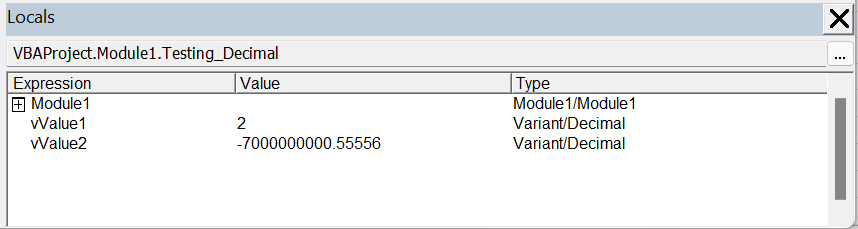# Variant/Decimal

The variant has a special sub type called Variant/Decimal that can be used to hold very large numbers.
This can support 29 significant figures with 28 decimal places.
This data type uses 14 bytes
The default value is Empty because it must be declared as Variant

### Possible Values

The smallest non zero value with 28 decimal places is:
+/- 0.0000000000 0000000000 000000001
The largest value with 28 decimal places is
+/- 7.9228162514 2643375935 43950335

`Dim vValue1 As Variant Dim vValue2 As Variant vValue1 = CDec(10 / 5) vValue2 = CDec(-7000000000.55556) Debug.Print VBA.TypeName(vValue1)  '= Decimal  Debug.Print VBA.TypeName(vValue2)  '= Decimal  `This data type must be declared as a Variant and can only be created using the CDEC conversion function.
Use this data type when you do not want rounding errors.
If you do not need this kind of precision you should always use the Double data type instead.

`Dim decMyDecimal As Variant decMyDecimal = CDec(3000000000000) Call MsgBox(TypeName(decMyDecimal) `

These are stored as 96 bit (12 byte) signed integers scaled by a variable power of 10.
The power of 10 scaling factor specifies the number of digits to the right of the decimal point and ranges from 0 to 28.
This data type provides the greatest number of significant digits for a number.
This data type is the slowest of all the numeric data types.
This data type widens to Single or Double
You can convert Decimal to Single or Double without an Overflow error.